Want to share your content on R-bloggers? click here if you have a blog, or here if you don't.

Andar, lo que es andar, anduve encima siempre de las nubes (Del tiempo perdido, Robe)

If you give importance to colours, maybe you know already COLOURlovers. As can be read in their website, COLOURlovers is a creative community where people from around the world create and share colors, palettes and patterns, discuss the latest trends and explore colorful articles… All in the spirit of love.

There is a R package called colourlovers which provides access to the COLOURlovers API. It makes very easy to choose nice colours for your graphics. I used clpalettes function to search for the top palettes of the website. Their names are pretty suggestive as well: Giant Goldfish, Thought Provoking, Adrift in Dreams, let them eat cake … Inspired by this post I have done a Shiny app to create colored flowers using that palettes. Seeds are arranged according to the golden angle. One example:Some others: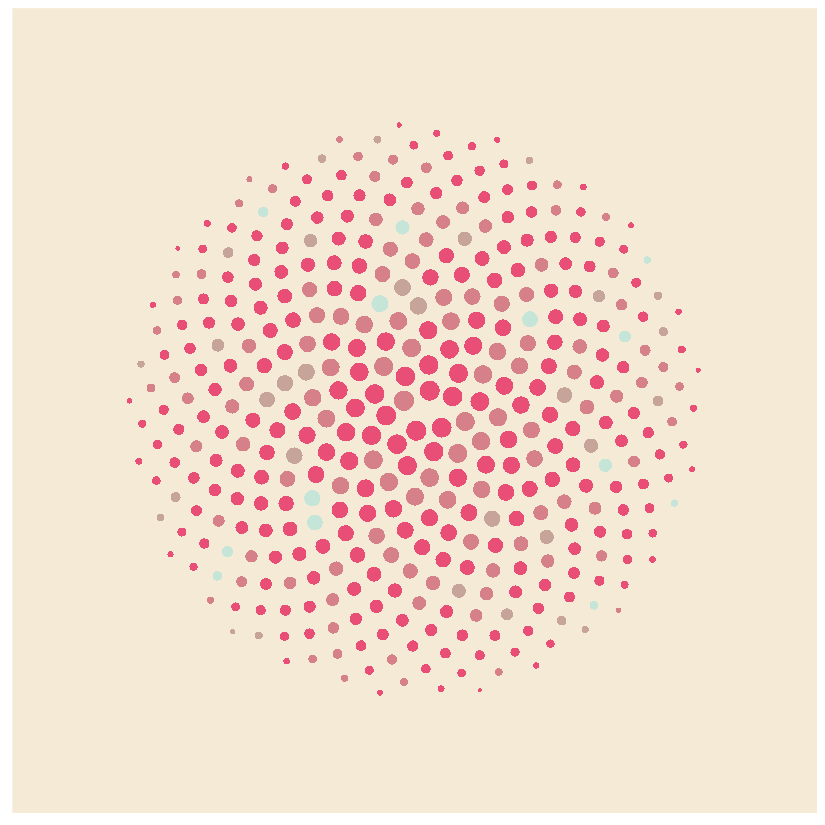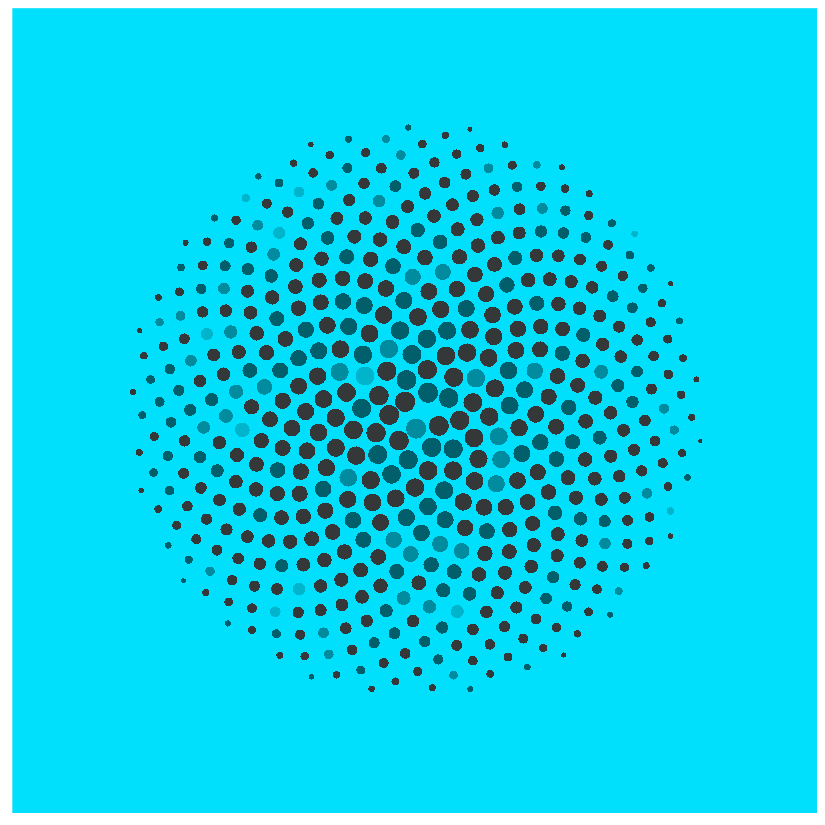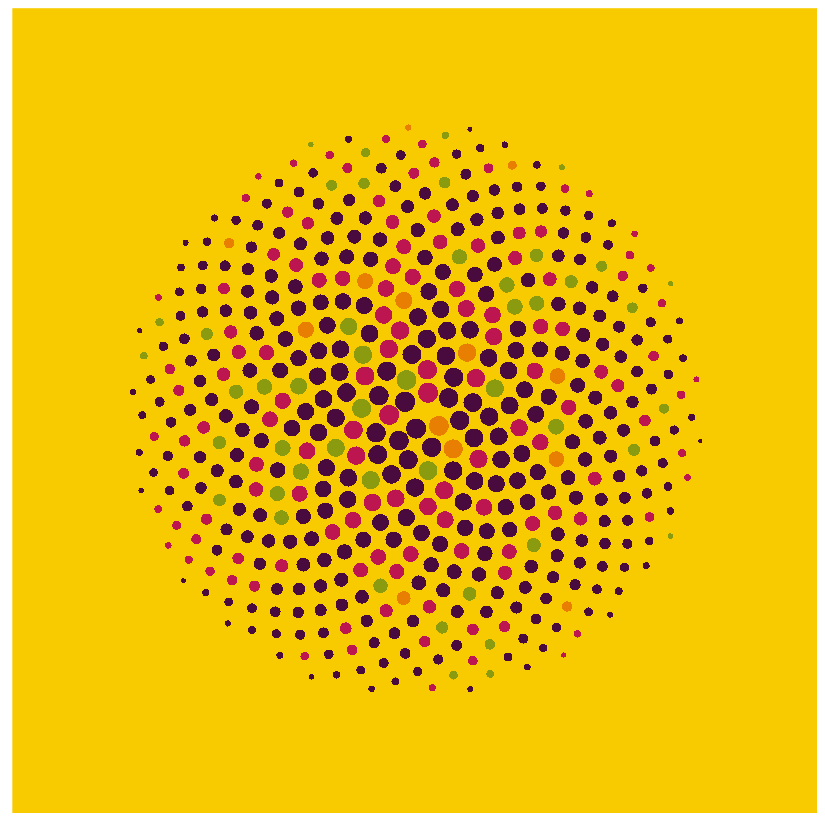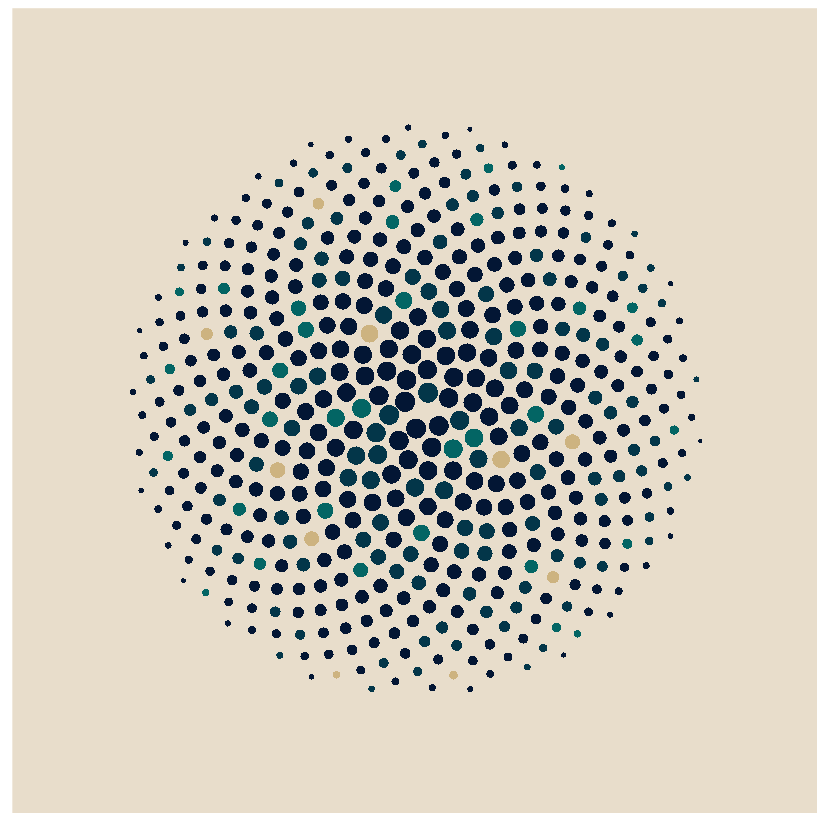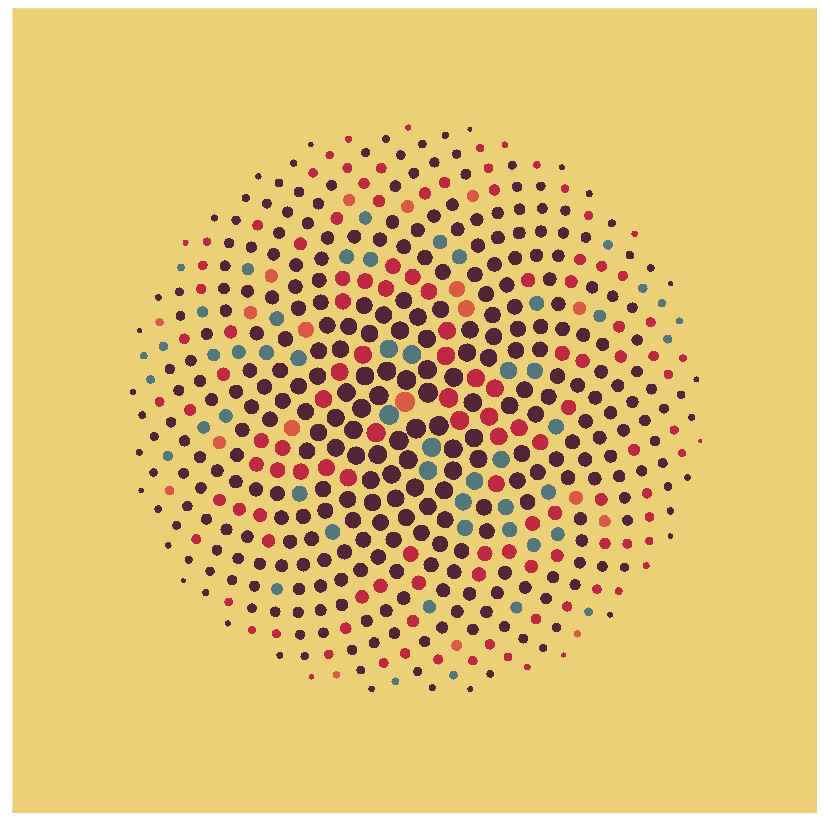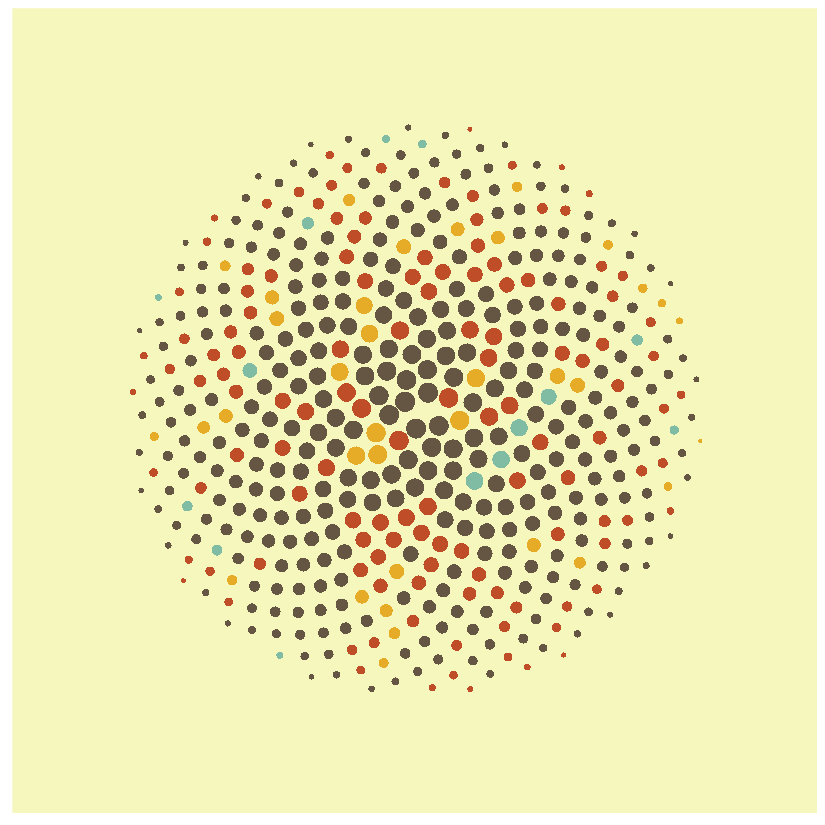You can play with the app here.

If you want to do your own sunflowers, here you have the code. This is the `ui.R` file:

```library(colourlovers)
library(rlist)
top=clpalettes('top')
sapply(1:length(top), function(x) list.extract(top, x)\$title)->titles

fluidPage(
titlePanel("Sunflowers for COLOURlovers"),
fluidRow(
column(3,
wellPanel(
selectInput("pal", label = "Palette:", choices = titles),
sliderInput("nob", label = "Number of points:", min = 200, max = 500, value = 400, step = 50)
)
),
mainPanel(
plotOutput("Flower")
)
)
)
```

And this is the `server.R` one:

```library(shiny)
library(ggplot2)
library(colourlovers)
library(rlist)
library(dplyr)

top=clpalettes('top')
sapply(1:length(top), function(x) list.extract(top, x)\$title)->titles

CreatePlot = function (ang=pi*(3-sqrt(5)), nob=150, siz=15, sha=21, pal="LoversInJapan") {

list.extract(top, which(titles==pal))\$colors %>%
unlist %>%
as.vector() %>%
paste0("#", .) -> all_colors

colors=data.frame(hex=all_colors, darkness=colSums(col2rgb(all_colors)))
colors %>% arrange(-darkness)->colors

background=colors[1,"hex"] %>% as.character

colors %>% filter(hex!=background) %>% .[,1] %>% as.vector()->colors

ggplot(data.frame(r=sqrt(1:nob), t=(1:nob)*ang*pi/180), aes(x=r*cos(t), y=r*sin(t)))+
geom_point(colour=sample(colors, nob, replace=TRUE, prob=exp(1:length(colors))), aes(size=(nob-r)), shape=16)+
scale_x_continuous(expand=c(0,0), limits=c(-sqrt(nob)*1.4, sqrt(nob)*1.4))+
scale_y_continuous(expand=c(0,0), limits=c(-sqrt(nob)*1.4, sqrt(nob)*1.4))+
theme(legend.position="none",
panel.background = element_rect(fill=background),
panel.grid=element_blank(),
axis.ticks=element_blank(),
axis.title=element_blank(),
axis.text=element_blank())}

function(input, output) {
output\$Flower=renderPlot({
CreatePlot(ang=180*(3-sqrt(5)), nob=input\$nob, siz=input\$siz, sha=as.numeric(input\$sha), pal=input\$pal)
}, height = 550, width = 550 )}
```